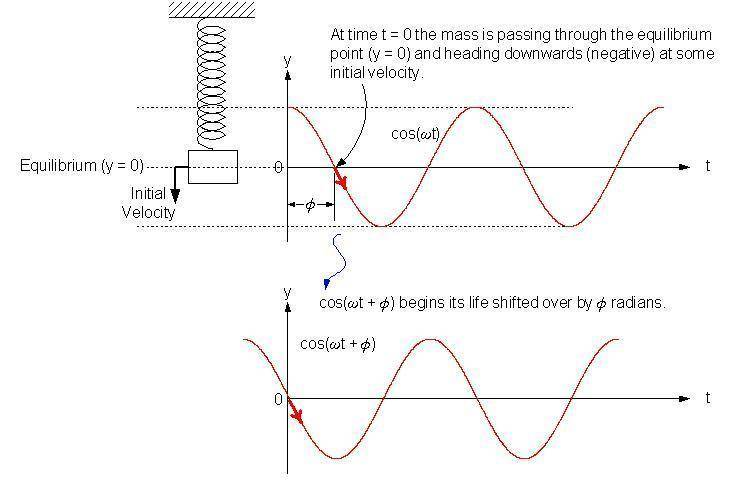# Phase constant of SHM

## Homework Statement

The displacement of a mass oscillating on a spring is given by x(t) = xmcos(ωt + ). If the initial displacement is zero and the initial velocity is in the negative x direction, then the phase constant is:

## The Attempt at a Solution

How do I start? The book just tells me that the phase constant depends on displacement and velocity when t = 0, but doesn't say how.

gneill
Mentor
Sketch a cosine curve. What's its initial value? Where on the curve would match the initial condition of the spring and mass? What's (angular) the offset from zero?

Sketch a cosine curve.

OK

What's its initial value?

1

Where on the curve would match the initial condition of the spring and mass?

Huh??

gneill
Mentor
Does the mass start at a maximum extension like the cosine function does?

No, initial displacement is 0. So, I need to find where cosx equals 0?

gneill
Mentor
No, initial displacement is 0. So, I need to find where cosx equals 0?

Not only that, but where it's going through zero and going negative, just like the mass' displacement.

Still have no clue on this.

gneill
Mentor
Have a gander: